Function Repository Resource:

# RandomPartition

Cut a list into random segments

Contributed by: Aster Ctor
 ResourceFunction["RandomPartition"][list,max] divides list into smaller pieces of length no more than max. ResourceFunction["RandomPartition"][list,min;;max] divides list into smaller pieces of length min to max. ResourceFunction["RandomPartition"][list,v] divides list into smaller pieces with lengths chosen from integer list v. ResourceFunction["RandomPartition"][list,p→v] divides list into smaller pieces with lengths chosen from list v according to weights p.

## Examples

### Basic Examples (1)

Divide a list of length 10 into smaller pieces of length no more than 4:

 In:=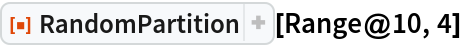Out=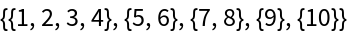### Scope (3)

Divide a list of length 10 into small pieces of lengths 2 to 4:

 In:=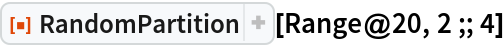Out=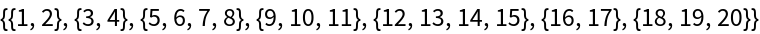Divide a list of length 10 into small pieces of length 2 or 4:

 In:=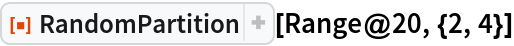Out=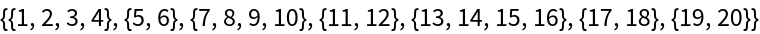Divide a list of length 10 into small blocks of length 2 or 4 according to the specified weights:

 In:=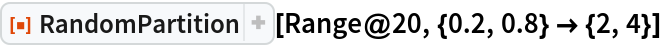Out=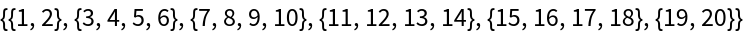### Possible Issues (1)

If the given set cannot be segmented as requested, the last elements will break the limit instead of being lost:

 In:=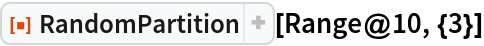Out=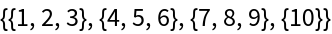## Publisher

Aster Ctor (MoeNet)

## Version History

• 1.0.0 – 26 December 2019